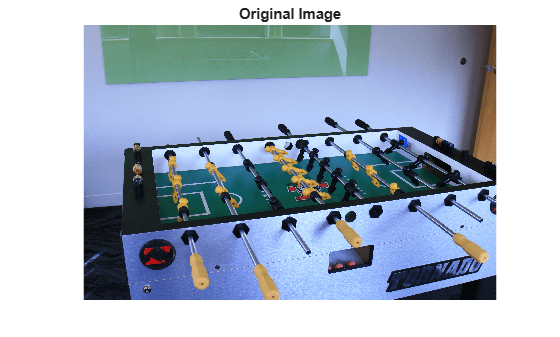# illumgray

Estimate illuminant using gray world algorithm

## Syntax

``illuminant = illumgray(A)``
``illuminant = illumgray(A,percentile)``
``illuminant = illumgray(___,Name,Value)``

## Description

````illuminant = illumgray(A)` estimates the illumination of the scene in RGB image `A` by assuming that the average color of the scene is gray.```

example

````illuminant = illumgray(A,percentile)` estimates the illumination, excluding the specified bottom and top percentiles of pixel values.```
````illuminant = illumgray(___,Name,Value)` estimates the illumination using name-value pairs to control additional options.```

## Examples

collapse all

Open and display an image with poor white balance.

```A = imread("foosball.jpg"); imshow(A) title("Original Image")```The gray world algorithm assumes that the RGB values are linear. However, the JPEG file format saves images in the gamma-corrected sRGB color space. Undo the gamma correction by using the `rgb2lin` function.

`A_lin = rgb2lin(A);`

Estimate the scene illumination, excluding the top and bottom 10% of pixels. Because the input image has been linearized, `illumgray` returns the illuminant in the linear RGB color space.

```percentiles = 10; illuminant = illumgray(A_lin,percentiles)```
```illuminant = 1×3 0.2206 0.2985 0.5219 ```

The third coefficient of `illuminant` is the largest, which is consistent with the blue tint of the image.

Correct colors by providing the estimated illuminant to the `chromadapt` function.

`B_lin = chromadapt(A_lin,illuminant,ColorSpace="linear-rgb");`

To display the white-balanced image correctly on the screen, apply gamma correction by using the `lin2rgb` function.

`B = lin2rgb(B_lin);`

Display the corrected image.

```imshow(B) title("White-Balanced Image Using Gray World with percentiles=["+ ... num2str(percentiles)+" "+num2str(percentiles)+"]")```## Input Arguments

collapse all

RGB image, specified as an m-by-n-by-3 numeric array.

Data Types: `single` | `double` | `uint8` | `uint16`

Percentile of pixels to exclude from the illuminant estimation, specified as a numeric scalar or 2-element numeric vector. Excluding pixels helps prevent overexposed and underexposed pixels from skewing the estimation.

• If `percentile` is a scalar, the same value is used for both the bottom percentile and the top percentile. In this case, `percentile` must be in the range [0, 50] so that the sum of the bottom and top percentiles does not exceed 100.

• If `percentile` is a 2-element vector, the first element is the bottom percentile and the second element is the top percentile. Both percentiles must be in the range [0, 100) and their sum cannot exceed 100.

The following image indicates the range of pixels that are included in the illuminant estimation. The selection is separate for each color channel.Data Types: `single` | `double` | `int8` | `int16` | `int32` | `int64` | `uint8` | `uint16` | `uint32` | `uint64`

### Name-Value Arguments

Specify optional pairs of arguments as `Name1=Value1,...,NameN=ValueN`, where `Name` is the argument name and `Value` is the corresponding value. Name-value arguments must appear after other arguments, but the order of the pairs does not matter.

Before R2021a, use commas to separate each name and value, and enclose `Name` in quotes.

Example: `illuminant = illumgray(I,'Mask',m)` estimates the scene illuminant using a subset of pixels in image `I`, selected according to a binary mask, `m`.

Image mask, specified as the comma-separated pair consisting of `'Mask'` and an m-by-n logical or numeric array. The mask indicates which pixels of the input image `A` to use when estimating the illuminant. The computation excludes pixels in `A` that correspond to a mask value of 0. By default, the mask has all 1s, and all pixels in `A` are included in the estimation.

Data Types: `single` | `double` | `int8` | `int16` | `int32` | `int64` | `uint8` | `uint16` | `uint32` | `uint64` | `logical`

Type of vector norm (p-norm), specified as the comma-separated pair consisting of `'Norm'` and a positive numeric scalar. The p-norm affects the calculation of the average RGB value in the input image `A`. The p-norm is defined as sum(abs(x)p) ^ (1/p).

Data Types: `single` | `double` | `int8` | `int16` | `int32` | `int64` | `uint8` | `uint16` | `uint32` | `uint64`

## Output Arguments

collapse all

Estimate of scene illumination, returned as a 3-element numeric row vector. The three elements correspond to the red, green, and blue values of the illuminant.

Data Types: `double`

## Tips

• The gray world algorithm assumes uniform illumination and linear RGB values. If you are working with nonlinear sRGB or Adobe RGB images, use the `rgb2lin` function to undo the gamma correction before using `illumgray`. Also, make sure to convert the chromatically adapted image back to sRGB by using the `lin2rgb` function.

• When you specify `Mask`, the bottom percentile and top percentile apply to the masked image.

• You can adjust the color balance of the image to remove the scene illumination by using the `chromadapt` function.

 Ebner, Marc. "The Gray World Assumption." Color Constancy. Chichester, West Sussex: John Wiley & Sons, 2007.